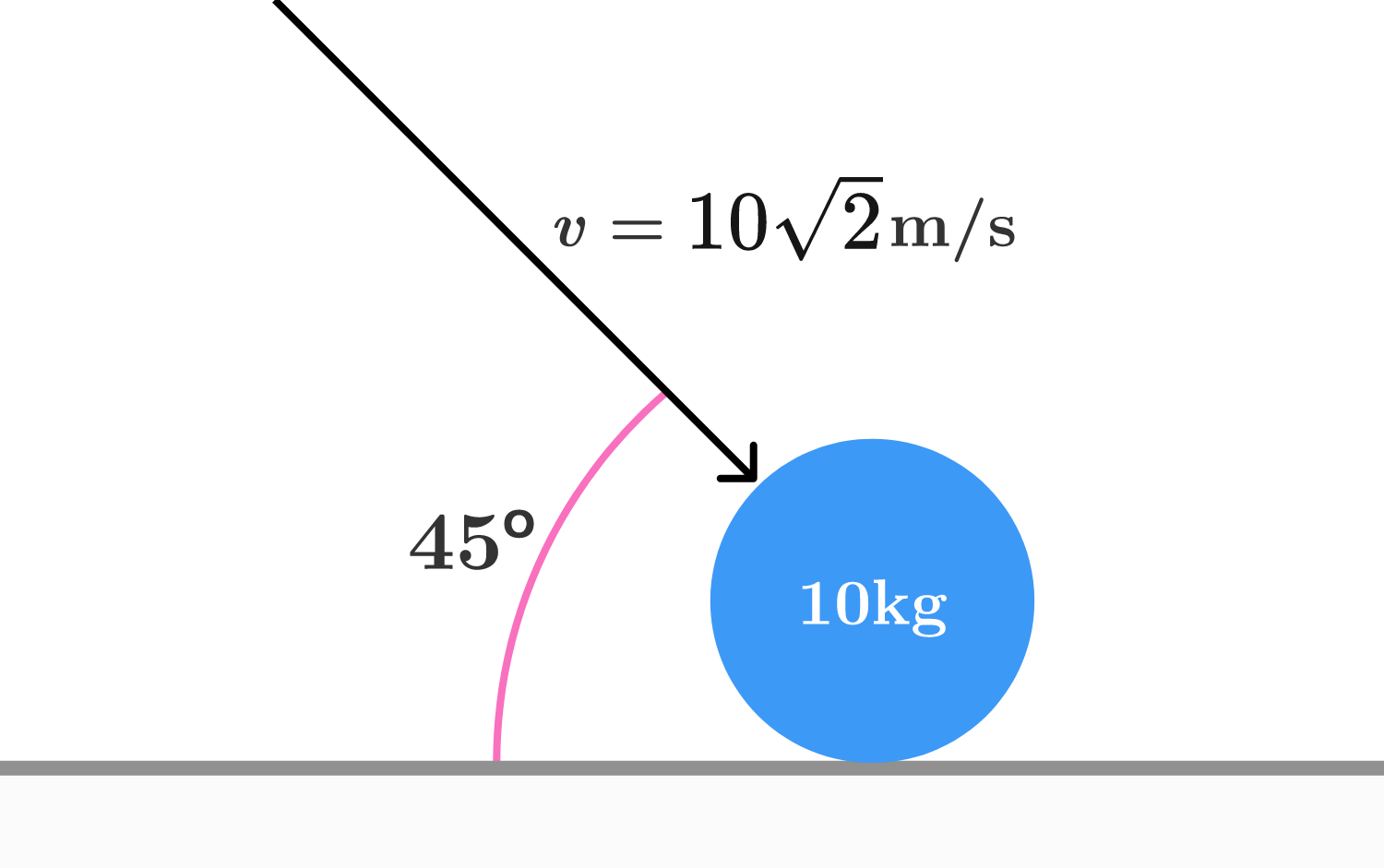# Ordinary scatter

A ball of mass $\SI{10}{\kilo\gram}$ hits a smooth horizontal surface with speed $\SI[per-mode=symbol]{10\sqrt 2}{\meter\per\second}$ at an angle of $45^\circ$. The coefficient of restitution between the ball and surface is $\epsilon = \SI{0.5}{}$ and the ball remains in contact with the surface for $\SI{0.1}{\second}$. Let $F$ be the instantaneous force exerted by the ball at any time during the collision.

Find the average value of the force, given by $\langle F \rangle$ in $\si{\newton}$.×